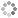# PROWAREtecharticles » current » dot-net » threading

How to use the Thread class in C#.

Working with threads in .NET is practically identical to using them in the Windows API. The end result is identical to Tasks except that they are implemented slightly differently.

Most asynchronous functions support the `CancellationToken` class so Tasks really are preferred. A thread can be cancelled through the use of a variable that is constantly being checked by the procedure. The same is happening with the `CancellationToken` but .NET `async` functions support the `CancellationToken`.

Threads seem to execute more quickly and in uniform order. Comparing Tasks performing the same operations, execution occurs in different order than the order in which they are created. This makes no difference for most apps.

### Creating and Starting a Thread

Using the .NET `Thread` class, threads can be created and controlled. After a `Thread` object is created, use the `Start()` method.

``````using System;

{
static void Main()
{
}
{
}
}
``````

Parameter(s) can be passed to the new thread's method but it must be passed as an `object` and then type cast to the original data type.

``````using System;

{
public struct Data
{
public string first;
public string last;
}
static void Main()
{
Data d;
d.first = "abc";
d.last = "xyz";

}
{
Data d2 = (Data)o;
Console.WriteLine("In a thread with parameter: " + d2.first);
}
}
``````

The application's process continues to run for as long as at least one foreground thread is running. If this is a problem then solve it by using background threads.

Specify the property `IsBackground = true` to create background threads. Notice: on Linux, threads that are not background ones are like separate processes and do not end when the creating process ends. How to kill a process on Linux.

``````using System;

{
static void Main()
{
}
{
}
}
``````

### Solve π on Each Logical Processor

This version of finding pi does so with integers. See this article for an example of using the .NET `Task` to find pi with floating point variables. `FindPi_2` is the popular Bailey–Borwein–Plouffe formula.

``````using System;
using System.Collections.Generic;
using System.Linq;

namespace ConsoleApp1
{
{
public int id { get; }
public ulong iterations { get; }
public double pi { get; set; }
public bool cancel { get; set; }
{
this.iterations = iterations;
this.id = id;
if((id & 1) == 0)
else
Console.WriteLine("Starting Job: {0, -" + Environment.ProcessorCount.ToString().Length + "} Iterations: {1}", id, iterations);
}

static void FindPi_1(object job) // this uses brute force to solve pi and it takes huge iterations to be as accurate as the formula below
{
//π = 3.14159265358979323846264338327950288419…
//π = (4/1) - (4/3) + (4/5) - (4/7) + (4/9) - (4/11) + (4/13) - (4/15) + ...
//π = 4 * (1 - 1/3 + 1/5 - 1/7 + 1/9 - 1/11 + 1/13 - 1/15 + ...)

long x = 1000000000000000000;
for (long i = 2; !((JobThread)job).cancel & i < count; i++)
x += ((i & 1) == 0 ? -1000000000000000000 : 1000000000000000000) / ((i << 1) - 1);
((JobThread)job).pi = 4 * (x / 1000000000000000000.0);
Console.WriteLine("Job: {0, -" + Environment.ProcessorCount.ToString().Length + "} ended with pi={1}", ((JobThread)job).id, ((JobThread)job).pi.ToString("0.00000000000000"));
}

static ulong Power(ulong x, ulong y)
{
ulong m = 1;
for (ulong i = 0; i < y; i++)
m *= x;
return m;
}
static void FindPi_2(object job) // this is the fast, efficient and accurate Bailey–Borwein–Plouffe formula
{
//π = 3.14159265358979323846264338327950288419...

ulong x = 0;
for (ulong k = 0, p = Power(16, k); (p > 0) & (k < count); k++, p = Power(16, k))
x += (4000000000000000000 / (8 * k + 1) - 2000000000000000000 / (8 * k + 4) - 1000000000000000000 / (8 * k + 5) - 1000000000000000000 / (8 * k + 6)) / p;
Console.WriteLine("Job: {0, -" + Environment.ProcessorCount.ToString().Length + "} ended with pi={1}", ((JobThread)job).id, ((JobThread)job).pi.ToString("0.00000000000000"));
}
}
class Program
{
static void Main(string[] args)
{
Console.WriteLine("pi={0}", 3.1415926535897932384626433832795028841971693993751M.ToString("0.00000000000000"));
Console.WriteLine("Logical Processors: {0}", Environment.ProcessorCount);
Console.WriteLine("ENTER A JOB NUMBER TO TERMINATE IT AT ANYTIME");

var spaces = Environment.ProcessorCount.ToString().Length;

int[] jobsIds = new int[Environment.ProcessorCount];
for (int i = 0; i < Environment.ProcessorCount; i++)
jobsIds[i] = i;

var rnd = new Random();
foreach (var jobId in jobsIds)
{
ulong count = (ulong)rnd.Next() * 10;
}

{
while (jobs.Where(j => j.thread.IsAlive == true).Count() > 0)
Environment.Exit(0);
})
{ IsBackground = true };

while (jobs.Where(j => j.thread.IsAlive == true).Count() > 0)
{This site uses cookies. Cookies are simple text files stored on the user's computer. They are used for adding features and security to this site. Read the privacy policy.